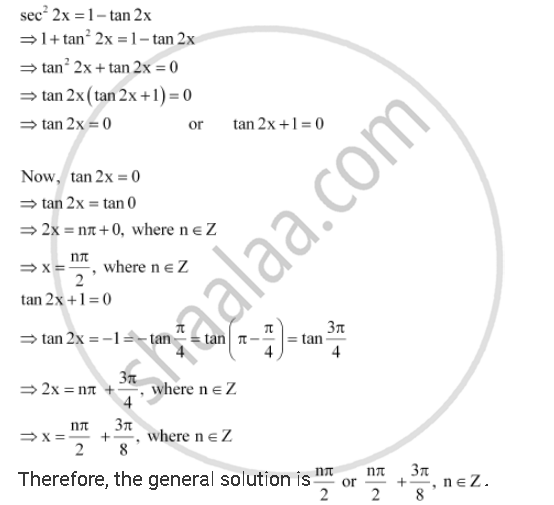CBSE (Arts) Class 11CBSE
Share

# Find the General Solution for Each of the Following Equations Sec2 2x = 1– Tan 2x - CBSE (Arts) Class 11 - Mathematics

ConceptTrigonometric Equations

#### Question

Find the general solution for each of the following equations sec2 2x = 1– tan 2x

#### SolutionIs there an error in this question or solution?

#### APPEARS IN

NCERT Solution for Mathematics Textbook for Class 11 (2018 to Current)
Chapter 3: Trigonometric Functions
Q: 8 | Page no. 78

#### Video TutorialsVIEW ALL 

Solution Find the General Solution for Each of the Following Equations Sec2 2x = 1– Tan 2x Concept: Trigonometric Equations.
S